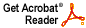# Examples of proper, closed, weakly dense subspaces in non-locally convex F-spaces

Joel H. Shapiro

Israel J. Math 7 (1969), 369--380

Abstract: I show that several classes of nonlocally convex F-spaces which admit separating families of continuous linear functionals also admit proper closed weakly dense subspaces (PCWD subspaces). V. L. Klee [Arch. Math. 7 (1956), 362--366] was the first to construct a topological linear space admitting a separating family of continuous linear functionals as well as a closed, non weakly closed subspace. N.T. Peck [Math. Ann. 161 (1965), 102--155] constructed a closed subspace of the above sort in the sequence space l^ p, 0 < p < 1; and P. L. Duren, B. W. Romberg and A. L. Shields [J. Reine Angew. Math. 238 (1969), 32--60] constructed PCWD subspaces in the Hardy spaces H^p, 0 < p < 1. In this paper I give a unified method to show that l^p, E(p, tau), and A^p (0 < p < 1) all admit PCWD subspaces. (E(p, tau) is the class of entire functions of exponential type tau with L^p restrictions to the real line, and A^p is the class of functions analytic in the open unit disc and in Lp there.) The essential step is to show that l^p is the continuous linear image of each of the latter two spaces.
Download .pdf file (328KB)